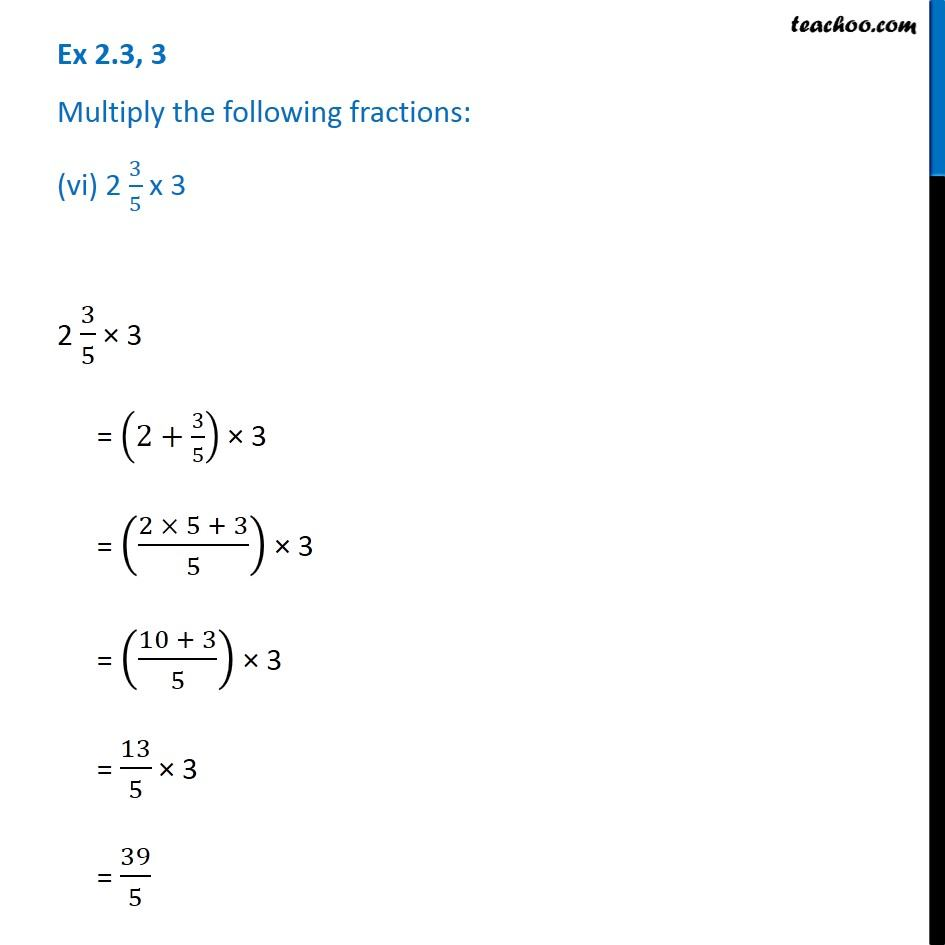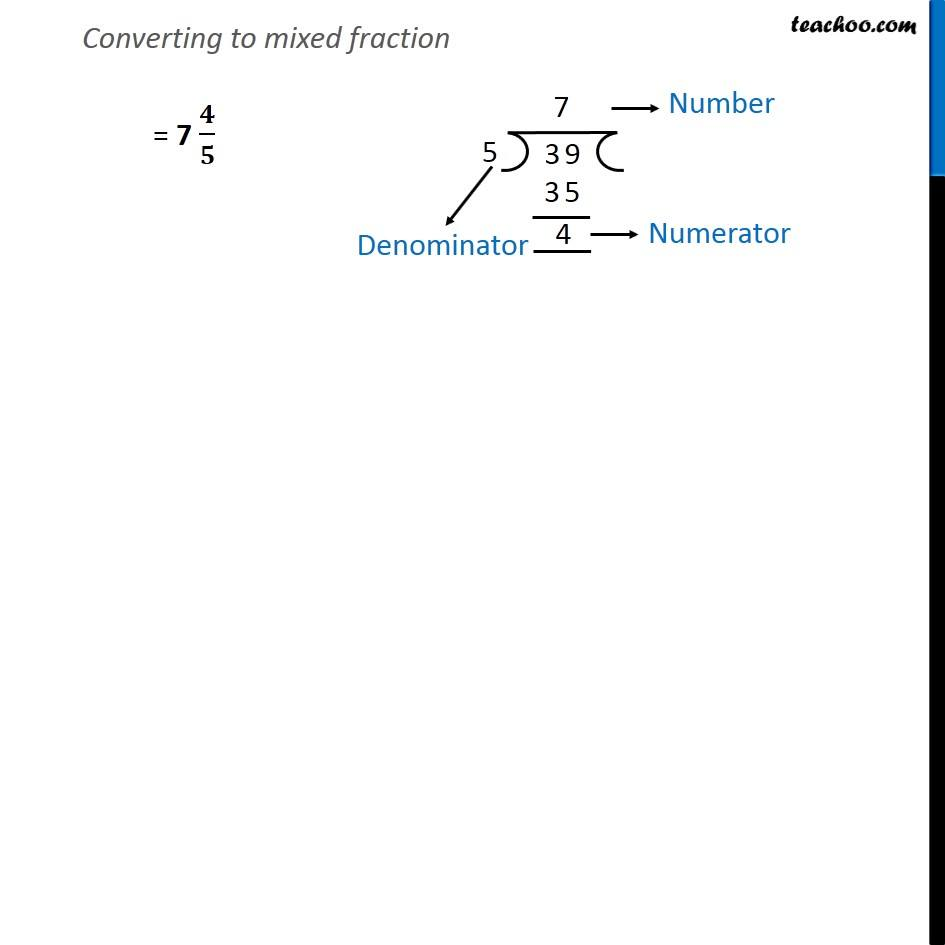Ex 2.2

Chapter 2 Class 7 Fractions and Decimals
Serial order wiseLearn in your speed, with individual attention - Teachoo Maths 1-on-1 Class

### Transcript

Ex 2.2, 3 Multiply the following fractions: (vi) 2 3/5 x 3 2 3/5 × 3 = (2+3/5) × 3 = ((2 × 5 + 3)/5) × 3 = ((10 + 3)/5) × 3 = 13/5 × 3 = 39/5 Converting to mixed fraction = 7 𝟒/𝟓